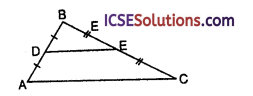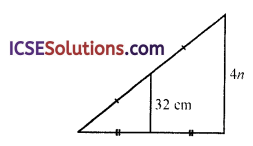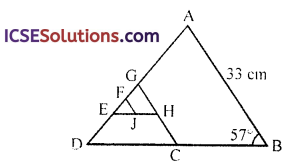Utilizing ICSE Class 9 Maths Solutions S Chand Chapter 9 Mid-Point and Intercept Theorems Chapter Test as a study aid can enhance exam preparation.

## S Chand Class 9 ICSE Maths Solutions Chapter 9 Mid-Point and Intercept Theorems Chapter Test

Question 1.
Use mid-segment theorem to name following parts of the given triangle.(a) A mid-segment of ∆ABC
(b) A segment parallel to AC
(c) A segment that has the same length as BD
(d) A segment that has half the length of AC
(e) A segment that has twice the length of EC
Solution:
In the given ∆ABC
AD = DB and BE = EC
∴ D and E are mid points of AB and BC respectively
∴ DE || AC and DE =$$\frac { 1 }{ 2 }$$ AC
(a) DE is mid segment of AABC
(b) DE is parallel to AC
(d) DE is half of AC
(e) Twice of EC = BC (∵ BE = EC)

Question 2.
Find each measure:
(a) NM
(b) XZ
(c) NZ
(d) ∠LMN
(e) ∠YXZ
(f) XXLMSolution:
In the given figure,
∵ YL = LX, YM = MZ and XN = NZ
∴ L, M and N are the mid points of sides XY,
YZ and XZ respectively
∴ LM = $$\frac { 1 }{ 2 }$$ XZ, MN = $$\frac { 1 }{ 2 }$$ XY
XY = 10 cm, LM = 6 cm, ∠MNZ = 25°‘
(a) NM = $$\frac { 1 }{ 2 }$$ XY = $$\frac { 1 }{ 2 }$$ x 10 cm = 5 cm

(b) XZ = 2 x LM = 2 x 6 = 12 cm

(c) NZ = $$\frac { 1 }{ 2 }$$ x XZ = $$\frac { 1 }{ 2 }$$ x 12 = 6 cm

(d) ∠LMN = 25°
(∵ LM || XZ and MN is a transversal)
(Alternate angles)

(e) ∠YXZ = ∠MNZ = 25°
(corresponding angles)

(f) ∠XLM = ∠XNM
(opposite angles of a ||gm) = 180° – 25° = 155°Question 3.
Find the value of n in each triangle.Solution:
In ∆ABC,AL = LB and CM = MB
∴ LM || AC and LM = $$\frac { 1 }{ 2 }$$ AC
⇒ 32 = $$\frac { 1 }{ 2 }$$ x 4n ⇒ 2n = 32 32
⇒ n = $$\frac { 32 }{ 2 }$$ = 16 cm
∴ n = 16 cm

Question 4.
Find the value of n in each triangle.Solution:
In ∆ABC,AE = EC and BD = DC
∴ DE || AB and DE = $$\frac { 1 }{ 2 }$$ AB
⇒ 6n = 4n + 12
⇒ 6n – 4n = +12
⇒ 2n = 12
n = $$\frac { 12 }{ 2 }$$ = 6

Question 5.
In the figure, CG, EH and FJ are mid-segments of ∆ABD, ∆GCD and ∆GHE respectively. Find each measure:
(a) CG
(b) EH
(c) FJ
(d) m∠DCG
(e) m∠GHE
(f) m∠FJHSolution:
In the given figure,
CG, EH and FJ are mid segments of ∆ABD, ∆GCD and ∆GHE respectively AB = 33 cm, ∠ABC = 57°
(a) CG = $$\frac { 1 }{ 2 }$$ AB
= $$\frac { 1 }{ 2 }$$ x 33 = 16.5 cm

(b) EH = $$\frac { 1 }{ 2 }$$ DC
= $$\frac { 1 }{ 2 }$$ x $$\frac { 1 }{ 2 }$$ DB
= $$\frac { 1 }{ 4 }$$ DB = $$\frac { 1 }{ 4 }$$ x 44 = 11 cm

(c) FJ = $$\frac { 1 }{ 2 }$$GH = $$\frac { 1 }{ 2 }$$ x $$\frac { 1 }{ 2 }$$ GC
= $$\frac { 1 }{ 4 }$$ GC = $$\frac { 1 }{ 4 }$$ x 6.5 cm = 4.125 cm

(d) m∠DCG = ∠DBA
(corresponding angles)
= 57°

(e) m∠GHE = ∠GCD
(corresponding angles)
= 57°

(f) m∠FJH = 180° – ∠GHE
= 180° – 57° = 123°Question 6.
Prove that the perimeter of a mid-segment triangle is half the perimeter of the triangle.
Solution:
In ∆ABC, D, E and F are the mid-points of sides BC, CA and AB respectively, forming mid segment ∆DEF∵ D, E and F are the mid points of the sides
∴ DE = $$\frac { 1 }{ 2 }$$ AB, EF = $$\frac { 1 }{ 2 }$$ BC and DF = $$\frac { 1 }{ 2 }$$ AC
Now perimeter of ∆DEF = DE + EF + DF
= $$\frac { 1 }{ 2 }$$AB + $$\frac { 1 }{ 2 }$$BC + $$\frac { 1 }{ 2 }$$CA
= $$\frac { 1 }{ 2 }$$(AB + BC + CA)
= Half perimeter of ∆ABC
Hence proved.